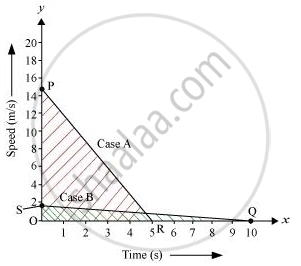# A driver of a car travelling at 52 km h^(−1) applies the brakes and accelerates uniformly in the opposite direction. The car stops in 5 s. - Science

A driver of a car travelling at 52 km h−1 applies the brakes and accelerates uniformly in the opposite direction. The car stops in 5 s. Another driver going at 3 km h−1 in another car applies his brakes slowly and stops in 10 s. On the same graph paper, plot the speed versus time graphs for the two cars. Which of the two cars travelled farther after the brakes were applied?

#### Solution

Case A:

Initial speed of the car, u1 = 52 km/h = 14.4 m/s

Time taken to stop the car, t1 = 5 s

Final speed of the car becomes zero after 5 s of application of brakes.

Case B:

Initial speed of the car, u2 = 3 km/h = 0.833 m/s ≅ 0.83 m/s

Time taken to stop the car, t2 = 10 s

Final speed of the car becomes zero after 10 s of application of brakes.

Plot of the two cars on a speed−time graph is shown in the following figure:-Distance covered by each car is equal to the area under the speed−time graph.

Distance covered in case A,

s_1=1/2xxOPxxOR=1/2xx14.4xx5=36

Distance covered in case B,

s_2=1/2xxOSxxOQ=1/2xx0.83xx10=4.15

Area of ΔOPR > Area of ΔOSQ

Thus, the distance covered in case A is greater than the distance covered in case B.

Hence, the car travelling with a speed of 52 km/h travels farther after brakes were applied.

Is there an error in this question or solution?

#### APPEARS IN

NCERT Class 9 Science Textbook
Chapter 8 Motion
Q 5 | Page 112